# swarmchart

Swarm scatter chart

Since R2020b

•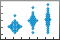## Syntax

``swarmchart(x,y)``
``swarmchart(x,y,sz)``
``swarmchart(x,y,sz,c)``
``swarmchart(___,mkr)``
``swarmchart(___,'filled')``
``swarmchart(tbl,xvar,yvar)``
``swarmchart(tbl,xvar,yvar,'filled')``
``swarmchart(ax,___)``
``swarmchart(___,Name,Value)``
``s = swarmchart(___)``

## Description

### Vector and Matrix Data

example

````swarmchart(x,y)` displays a swarm chart, which is a scatter plot with the points offset (jittered) in the `x`-dimension. The points form distinct shapes, and the outline of each shape is similar to a violin plot. Swarm charts help you to visualize discrete `x` data with the distribution of the `y` data. At each location in `x`, the points are jittered based on the kernel density estimate of `y`. To plot one set of points, specify `x` and `y` as vectors of equal length.To plot multiple sets of points on the same set of axes, specify at least one of `x` or `y` as a matrix. ```

example

````swarmchart(x,y,sz)` specifies the marker sizes. To plot all the markers with the same size, specify `sz` as a scalar. To plot the markers with different sizes, specify `sz` as a vector or a matrix.```

example

````swarmchart(x,y,sz,c)` specifies the marker colors. You can specify one color for all the markers, or you can vary the color. For example, you can plot all red circles by specifying `c` as `'red'`.```

example

````swarmchart(___,mkr)` specifies a different marker than the default marker, which is a circle. Specify `mkr` after all the arguments in any of the previous syntaxes.```

example

````swarmchart(___,'filled')` fills in the markers. Specify the `'filled'` option after all the arguments in any of the previous syntaxes.```

### Table Data

example

````swarmchart(tbl,xvar,yvar)` plots the variables `xvar` and `yvar` from the table `tbl`. To plot one data set, specify one variable for `xvar` and one variable for `yvar`. To plot multiple data sets, specify multiple variables for `xvar`, `yvar`, or both. If both arguments specify multiple variables, they must specify the same number of variables.```

example

````swarmchart(tbl,xvar,yvar,'filled')` plots the specified variables and fills in the markers.```

example

````swarmchart(ax,___)` displays the swarm chart in the target axes. Specify the axes before all the arguments in any of the previous syntaxes.```

example

````swarmchart(___,Name,Value)` specifies additional properties for the swarm chart using one or more name-value arguments. Specify the properties after all other input arguments. For example: `swarmchart(x,y,'LineWidth',2)` creates a swarm chart with 2-point marker outlines.`swarmchart(tbl,'MyX','MyY','ColorVariable','MyColors')` creates a swarm chart from data in a table, and customizes the marker colors using data from the table.`swarmchart(x,y,'YJitter','density')` creates a horizontal swarm chart. (since R2023b) For a list of properties, see Scatter Properties.```

example

````s = swarmchart(___)` returns the `Scatter` object or an array of `Scatter` objects. Use `s` to modify properties of the chart after creating it. For a list of properties, see Scatter Properties.```

## Examples

collapse all

Create a vector of `x` coordinates, and use the `randn` function to generate normally distributed random values for `y`. Then create a swarm chart of `x` and `y`.

```x = [ones(1,500) 2*ones(1,500) 3*ones(1,500)]; y1 = 2 * randn(1,500); y2 = 3 * randn(1,500) + 5; y3 = 5 * randn(1,500) + 5; y = [y1 y2 y3]; swarmchart(x,y)```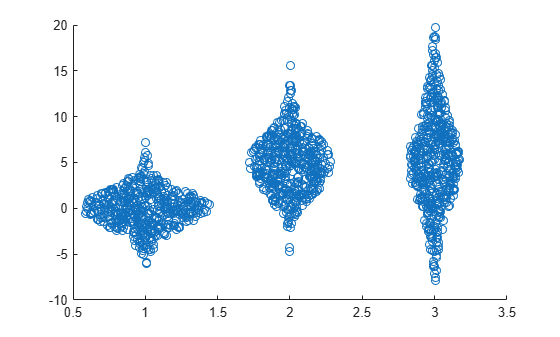Create three sets of `x` and `y` coordinates. Use the `randn` function to generate random values for `y`.

```x1 = ones(1,500); x2 = 2 * ones(1,500); x3 = 3 * ones(1,500); y1 = 2 * randn(1,500); y2 = [randn(1,250) randn(1,250) + 4]; y3 = 5 * randn(1,500) + 5;```

Create a swarm chart of the first data set, and specify a uniform marker size of `5`. Then call `hold on` to plot the second and third data sets together with the first data set. Call `hold off` to release the hold state of the axes.

```swarmchart(x1,y1,5) hold on swarmchart(x2,y2,5) swarmchart(x3,y3,5) hold off```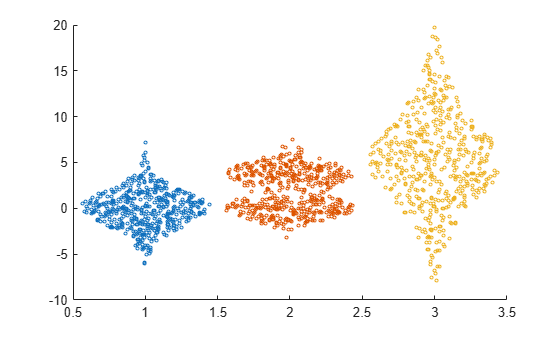Read the `BicycleCounts.csv` data set into a timetable called `tbl`. This data set contains bicycle traffic data over a period of time. Display the first five rows of `tbl`.

```tbl = readtable("BicycleCounts.csv"); tbl(1:5,:)```
```ans=5×5 table Timestamp Day Total Westbound Eastbound ____________________ _____________ _____ _________ _________ 24-Jun-2015 00:00:00 {'Wednesday'} 13 9 4 24-Jun-2015 01:00:00 {'Wednesday'} 3 3 0 24-Jun-2015 02:00:00 {'Wednesday'} 1 1 0 24-Jun-2015 03:00:00 {'Wednesday'} 1 1 0 24-Jun-2015 04:00:00 {'Wednesday'} 1 1 0 ```

Create a vector `x` with the day name from each observation, and another vector y with the bicycle traffic observed. Then create a swarm chart of `x` and `y`, and specify the point marker `('.')`. The chart shows the distribution of bicycle traffic according to the day of the week.

```daynames = ["Sunday" "Monday" "Tuesday" "Wednesday" "Thursday" "Friday" "Saturday"]; x = categorical(tbl.Day,daynames); y = tbl.Total; swarmchart(x,y,'.');```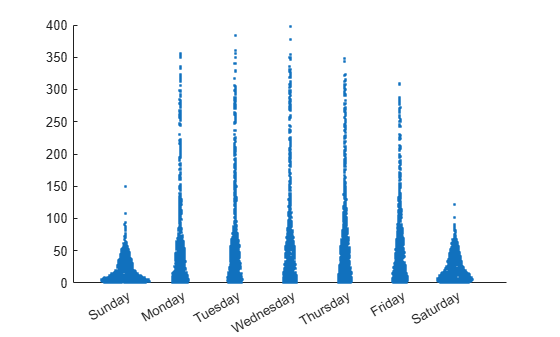Read the `BicycleCounts.csv` data set into a timetable called `tbl`. Create a vector `x` with the day name for each observation, another vector `y` with the bicycle traffic observed, and a third vector `c` with the hour of the day.

Then create a swarm chart of `x` and `y`, and specify the marker size as `20`. Specify the colors of the markers as vector `c`. The values in the vector index into the figure's colormap. Thus, the colors change according to the hour for each data point. Use the `'filled'` option to fill the markers with color instead of displaying them as hollow circles.

```tbl = readtable("BicycleCounts.csv"); daynames = ["Sunday" "Monday" "Tuesday" "Wednesday" "Thursday" "Friday" "Saturday"]; x = categorical(tbl.Day,daynames); y = tbl.Total; c = hour(tbl.Timestamp); swarmchart(x,y,20,c,'filled');```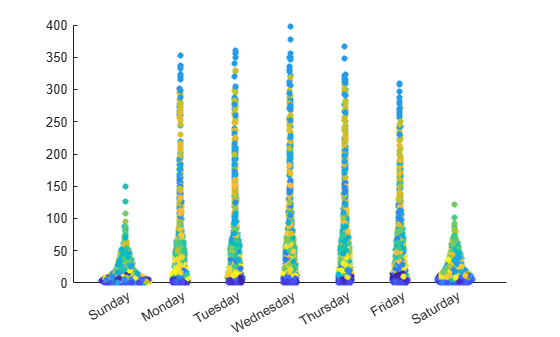Read the `BicycleCounts.csv` data set into a timetable called `tbl`. Create a vector `x` with the day name for each observation, another vector `y` with the bicycle traffic observed, and a third vector `c` with the hour of the day. Then create a swarm chart of `x` and `y`, and specify the marker size as `5`, and the colors of the markers as vector `c`. Call the `swarmchart` function with the return argument `s`, so that you can modify the chart after creating it.

```tbl = readtable("BicycleCounts.csv"); daynames = ["Sunday" "Monday" "Tuesday" "Wednesday" "Thursday" "Friday" "Saturday"]; x = categorical(tbl.Day,daynames); y = tbl.Total; c = hour(tbl.Timestamp); s = swarmchart(x,y,5,c);```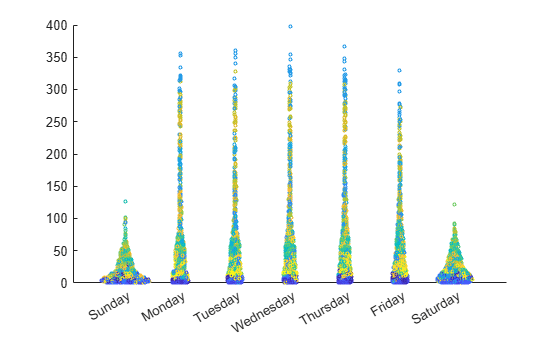Change the shapes of the clusters at each `x` location, so that the points are uniformly and randomly distributed and the spacing is limited to no more than `0.5` data units.

```s.XJitter = 'rand'; s.XJitterWidth = 0.5;```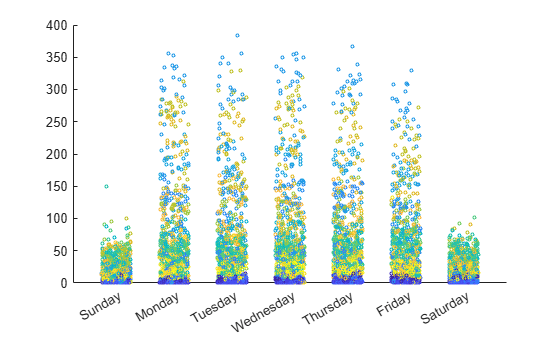Since R2023b

You can create a horizontal swarm chart by setting the `YJitter` property when you call the `swarmchart` function.

For example, create three normal distributions of 500 numbers and a categorical vector of town names. Then create a horizontal swarm chart of the data by calling the `swarmchart` function and specifying the `YJitter` name-value argument.

```x = randn(500,3) + [1 4 6]; towns = categorical(["Stowe" "Wayland" "Natick"]); y = repmat(towns,500,1); swarmchart(x,y,YJitter="density")```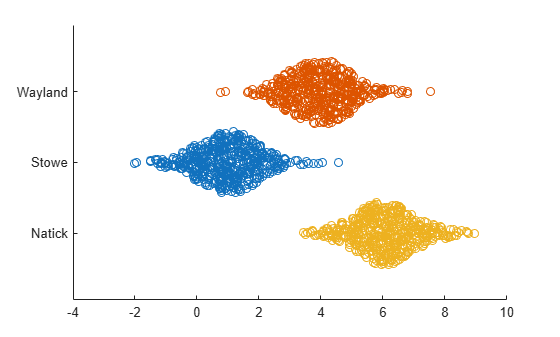Create a pair of `x` and `y` coordinates. Use the `randn` function to generate random values for `y`. Then create a swarm chart with filled markers that are 50% transparent both on their faces and on their edges.

```x1 = ones(1,500); x2 = 2 * ones(1,500); x = [x1 x2]; y1 = 2 * randn(1,500); y2 = [randn(1,250) randn(1,250) + 4]; y = [y1 y2]; swarmchart(x,y,'filled','MarkerFaceAlpha',0.5,'MarkerEdgeAlpha',0.5)```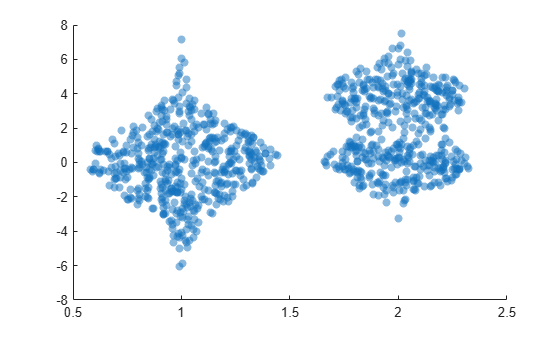A convenient way to plot data from a table is to pass the table to the `swarmchart` function and specify the variables you want to plot. For example, create a table with three variables of random numbers, and plot the `X` and `Y1` variables. By default, the axis labels match the variable names.

```tbl = table(randi(2,100,1),randn(100,1),randn(100,1)+10, ... 'VariableNames',{'X','Y1','Y2'}); swarmchart(tbl,'X','Y1')```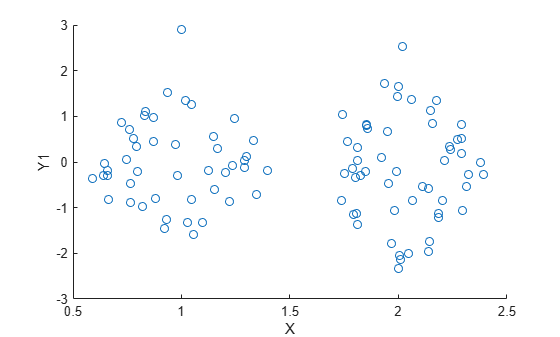You can also plot multiple variables at the same time. For example, plot the `Y1` and `Y2` variables on the y-axis by specifying the `yvar` argument as the cell array `{'Y1','Y2'}`. Then add a legend. The legend labels match the variable names.

```swarmchart(tbl,'X',{'Y1','Y2'}) legend```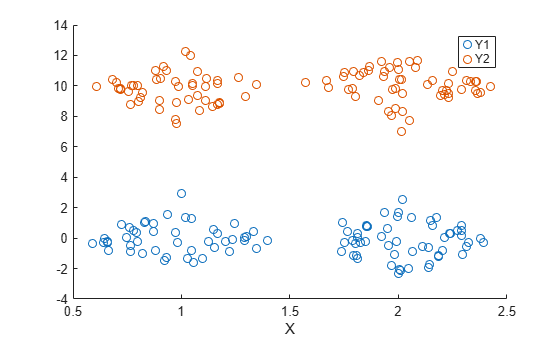One way to plot data from a table and customize the colors and marker sizes is to set the `ColorVariable` and `SizeData` properties. You can set these properties as name-value arguments when you call the `swarmchart` function, or you can set them on the `Scatter` object later.

For example, create a table with three variables of random numbers, and plot the `X` and `Y` variables with filled markers. Vary the marker colors by specifying the `ColorVariable` name-value argument. Return the `Scatter` object as `s`, so you can set other properties later.

```tbl = table(randi(2,100,1),randn(100,1),randn(100,1), ... 'VariableNames',{'X','Y','Colors'}); s = swarmchart(tbl,'X','Y','filled','ColorVariable','Colors');```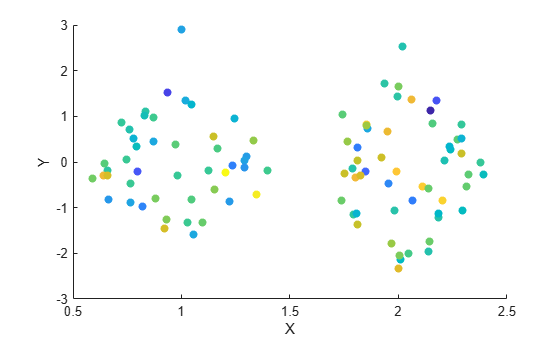Change the marker sizes to 100 points by setting the `SizeData` property.

`s.SizeData = 100;`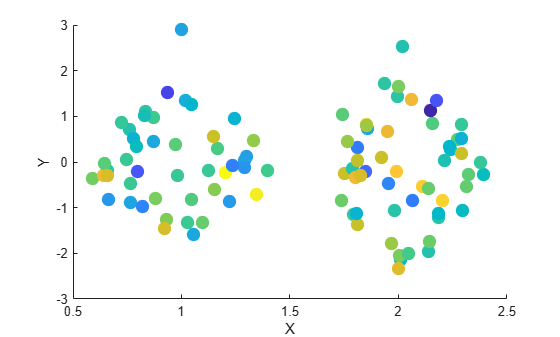Read the `BicycleCounts.csv` data set into a timetable called `tbl`. This data set contains bicycle traffic data over a period of time. Display the first five rows of `tbl`.

```tbl = readtable("BicycleCounts.csv"); tbl(1:5,:)```
```ans=5×5 table Timestamp Day Total Westbound Eastbound ____________________ _____________ _____ _________ _________ 24-Jun-2015 00:00:00 {'Wednesday'} 13 9 4 24-Jun-2015 01:00:00 {'Wednesday'} 3 3 0 24-Jun-2015 02:00:00 {'Wednesday'} 1 1 0 24-Jun-2015 03:00:00 {'Wednesday'} 1 1 0 24-Jun-2015 04:00:00 {'Wednesday'} 1 1 0 ```

Define `x` as a categorical array of the day names in the table. Define `yEast` and `yWest` as vectors containing the eastbound and westbound bicycle traffic counts.

```daynames = ["Sunday" "Monday" "Tuesday" "Wednesday" "Thursday" "Friday" "Saturday"]; x = categorical(tbl.Day,daynames); yEast = tbl.Eastbound; yWest = tbl.Westbound;```

Create a tiled chart layout in the `'flow'` tile arrangement, so that the axes fill the available space in the layout. Call the `nexttile` function to create an axes object and return it as `ax1`. Then create a swarm chart of the eastbound data by passing `ax1` to the `swarmchart` function.

```tiledlayout('flow') ax1 = nexttile; y = tbl.Eastbound; swarmchart(ax1,x,y,'.')```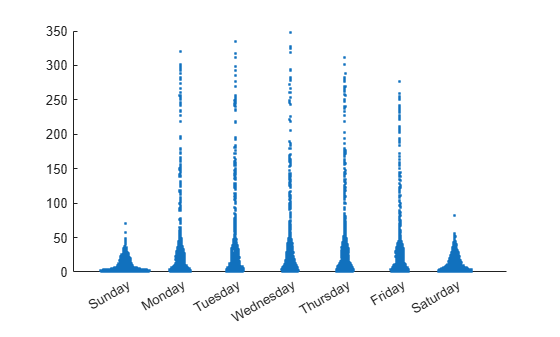Repeat the process to create a second axes object and a swarm chart for the westbound traffic.

```ax2 = nexttile; y = tbl.Westbound; swarmchart(ax2,x,y,'.')```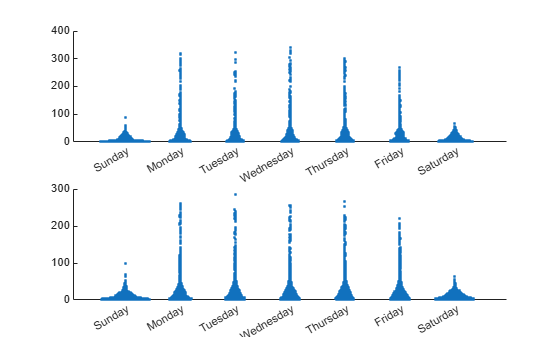## Input Arguments

collapse all

x-coordinates, specified as a scalar, vector, or matrix. The size and shape of `x` depends on the shape of your data. This table describes the most common situations.

Type of PlotHow to Specify Coordinates
Single point

Specify `x` and `y` as scalars. For example:

`swarmchart(1,1)`

One set of points

Specify `x` and `y` as any combination of row or column vectors of the same length. For example:

```x = randi(3,100,1); y = randn(1,100); swarmchart(x,y)```

Multiple sets of points that are different colors

If all the sets share the same x- or y-coordinates, specify the shared coordinates as a vector and the other coordinates as a matrix. The length of the vector must match one of the dimensions of the matrix. For example:

```x = randi(2,1,100); y = [randn(100,1) randn(100,1)+5]; swarmchart(x,y,100)```
If the matrix is square, `swarmchart` plots a separate set of points for each column in the matrix.

Alternatively, specify `x` and `y` as matrices of equal size. In this case, `swarmchart` plots each column of `y` against the corresponding column of `x`. For example:

```x = randi(2,100,2); y = [randn(100,1) randn(100,1)+5]; swarmchart(x,y,100)```

Data Types: `single` | `double` | `int8` | `int16` | `int32` | `int64` | `uint8` | `uint16` | `uint32` | `uint64` | `categorical`

y-coordinates, specified as a scalar, vector, or matrix. The size and shape of `y` depends on the shape of your data. This table describes the most common situations.

Type of PlotHow to Specify Coordinates
Single point

Specify `x` and `y` as scalars. For example:

`swarmchart(1,1)`

One set of points

Specify `x` and `y` as any combination of row or column vectors of the same length. For example:

```x = randi(3,100,1); y = randn(1,100); swarmchart(x,y)```

Multiple sets of points that are different colors

If all the sets share the same x- or y-coordinates, specify the shared coordinates as a vector and the other coordinates as a matrix. The length of the vector must match one of the dimensions of the matrix. For example:

```x = randi(2,1,100); y = [randn(100,1) randn(100,1)+5]; swarmchart(x,y,100)```
If the matrix is square, `swarmchart` plots a separate set of points for each column in the matrix.

Alternatively, specify `x` and `y` as matrices of equal size. In this case, `swarmchart` plots each column of `y` against the corresponding column of `x`. For example:

```x = randi(2,100,2); y = [randn(100,1) randn(100,1)+5]; swarmchart(x,y,100)```

Data Types: `single` | `double` | `int8` | `int16` | `int32` | `int64` | `uint8` | `uint16` | `uint32` | `uint64` | `categorical` | `datetime` | `duration`

Marker size, specified as a numeric scalar, vector, matrix, or empty array (`[]`). The size controls the area of each marker in points squared. An empty array specifies the default size of 36 points. The way you specify the size depends on how you specify `x` and `y`, and how you want the plot to look. This table describes the most common situations.

Desired Marker Sizes`x` and `y` `sz`Example

Same size for all points

Any valid combination of vectors or matrices described for `x` and `y`.

Scalar

Specify `x` as a vector, `y` as a matrix, and `sz` as a scalar.

```x = randi(2,1,100); y = randn(100,1); swarmchart(x,y,100)```

Different size for each point

Vectors of the same length

• A vector with the same length as `x` and `y`.

• A matrix with at least one dimension that matches the lengths of `x` and `y`. Specifying a matrix is useful for displaying multiple markers with different sizes at each (x,y) location.

Specify `x`, `y`, and `sz` as vectors.

```x = randi(2,1,100); y = randn(100,1); sz = randi([70 2000],100,1); swarmchart(x,y,sz)```

Specify `x` and `y` as vectors and `sz` as a matrix.

```x = randi(2,1,100); y = randn(100,1); sz = randi([70 2000],100,2); swarmchart(x,y,sz)```

Different size for each point

At least one of `x` or `y` is a matrix for plotting multiple data sets

• A vector with the same number of elements as there are points in each data set.

• A matrix that has the same size as the `x` or `y` matrix.

Specify `x` as a vector, `y` as a matrix, and `sz` as vector.

```x = randi(2,1,100); y = [randn(100,1) randn(100,1)+5]; sz = randi([70 2000],100,1); swarmchart(x,y,sz)```

Specify `x` as a vector, `y` as a matrix, and `sz` as a matrix the same size as `y`.

```x = randi(2,1,100); y = [randn(100,1) randn(100,1)+5]; sz = randi([70 2000],100,2); swarmchart(x,y,sz)```

Marker color, specified as a color name, RGB triplet, matrix of RGB triplets, or a vector of colormap indices.

• Color name — A color name such as `'red'`, or a short name such as `'r'`.

• RGB triplet — A three-element row vector whose elements specify the intensities of the red, green, and blue components of the color. The intensities must be in the range `[0,1]`; for example, ```[0.4 0.6 0.7]```. RGB triplets are useful for creating custom colors.

• Matrix of RGB triplets — A three-column matrix in which each row is an RGB triplet.

• Vector of colormap indices — A vector of numeric values that is the same length as the `x` and `y` vectors.

The way you specify the color depends on the desired color scheme and whether you are plotting one set of coordinates or multiple sets of coordinates. This table describes the most common situations.

Color SchemeHow to Specify the ColorExample

Use one color for all the points.

Specify a color name or a short name from the table below, or specify one RGB triplet.

Plot one set of points, and specify the color as `'red'`.

```x = randi(2,1,100); y = randn(100,1); c = 'red'; swarmchart(x,y,[],c)```

Plot two sets of points, and specify the color as red using an RGB triplet.

```x = randi(2,1,100); y = randn(100,1); c = [0.6 0 0.9]; swarmchart(x,y,[],c)```

Assign different colors to each point using a colormap.

Specify a row or column vector of numbers. The numbers index into the current colormap array. The smallest value maps to the first row in the colormap, and the largest value maps to the last row. The intermediate values map linearly to the intermediate rows.

If your plot has three points, specify a column vector to ensure the values are interpreted as colormap indices.

You can use this method only when `x`, `y`, and `sz` are all vectors.

Create a vector `c` that specifies 100 colormap indices. Plot 100 points using the colors from the current colormap. Then, change the colormap to `winter`.

```x = randi(2,1,100); y = randn(100,1); c = 1:100; swarmchart(x,y,[],c) colormap(gca,'winter')```

Create a custom color for each point.

Specify an m-by-3 matrix of RGB triplets, where m is the number of points in the plot.

You can use this method only when `x`, `y`, and `sz` are all vectors.

Create a matrix `c` that specifies 100 random RGB triplets. Then create a swarm chart of 100 points using those colors.

```x = randi(2,1,100); y = randn(100,1); c = rand(100,3); swarmchart(x,y,[],c)```

Create a different color for each data set.

Specify an n-by-3 matrix of RGB triplets, where n is the number of data sets.

You can use this method only when at least one of `x`, `y`, or `sz` is a matrix.

Create a matrix `c` that contains two RGB triplets. Then plot two data sets using those colors.

```x = randi(2,100,2); y = [randn(100,1) randn(100,1)+5]; c = [1 0 0; 0 0 1]; swarmchart(x,y,[],c)```

#### Color Names and RGB Triplets for Common Colors

Color NameShort NameRGB TripletHexadecimal Color CodeAppearance
`"red"``"r"``[1 0 0]``"#FF0000"``"green"``"g"``[0 1 0]``"#00FF00"``"blue"``"b"``[0 0 1]``"#0000FF"``"cyan"` `"c"``[0 1 1]``"#00FFFF"``"magenta"``"m"``[1 0 1]``"#FF00FF"``"yellow"``"y"``[1 1 0]``"#FFFF00"``"black"``"k"``[0 0 0]``"#000000"``"white"``"w"``[1 1 1]``"#FFFFFF"`Here are the RGB triplets and hexadecimal color codes for the default colors MATLAB® uses in many types of plots.

`[0 0.4470 0.7410]``"#0072BD"``[0.8500 0.3250 0.0980]``"#D95319"``[0.9290 0.6940 0.1250]``"#EDB120"``[0.4940 0.1840 0.5560]``"#7E2F8E"``[0.4660 0.6740 0.1880]``"#77AC30"``[0.3010 0.7450 0.9330]``"#4DBEEE"``[0.6350 0.0780 0.1840]``"#A2142F"`Marker type, specified as one of the values listed in this table.

MarkerDescriptionResulting Marker
`"o"`Circle`"+"`Plus sign`"*"`Asterisk`"."`Point`"x"`Cross`"_"`Horizontal line`"|"`Vertical line`"square"`Square`"diamond"`Diamond`"^"`Upward-pointing triangle`"v"`Downward-pointing triangle`">"`Right-pointing triangle`"<"`Left-pointing triangle`"pentagram"`Pentagram`"hexagram"`HexagramOption to fill the interior of the markers, specified as `'filled'`. Use this option with markers that have a face, for example, `'o'` or `'square'`. Markers that do not have a face and contain only edges do not render at all (`'+'`, `'*'`, `'.'`, and `'x'`).

The `'filled'` option sets the `MarkerFaceColor` property of the `Scatter` object to `'flat'` and the `MarkerEdgeColor` property to `'none'`. In this case, MATLAB draws the marker faces, but not the edges.

Source table containing the data to plot, specified as a table or a timetable.

Table variables containing the x-coordinates, specified as one or more table variable indices.

#### Specifying Table Indices

Use any of the following indexing schemes to specify the desired variable or variables.

Indexing SchemeExamples

Variable names:

• `"A"` or `'A'` — A variable named `A`

• `["A","B"]` or `{'A','B'}` — Two variables named `A` and `B`

• `"Var"+digitsPattern(1)` — Variables named `"Var"` followed by a single digit

Variable index:

• An index number that refers to the location of a variable in the table.

• A vector of numbers.

• A logical vector. Typically, this vector is the same length as the number of variables, but you can omit trailing `0` or `false` values.

• `3` — The third variable from the table

• `[2 3]` — The second and third variables from the table

• `[false false true]` — The third variable

Variable type:

• `vartype("categorical")` — All the variables containing categorical values

The table variables you specify can contain numeric or categorical values.

To plot one data set, specify one variable for `xvar` and one variable for `yvar`. For example, create a table with three variables of normally distributed random values. Plot the `X1` and `Y` variables.

```tbl = table(randn(100,1),randn(100,1)+10,randn(100,1), ... 'VariableNames',{'X1','X2','Y'}); swarmchart(tbl,'X1','Y')```

To plot multiple data sets together, specify multiple variables for `xvar`, `yvar`, or both. If you specify multiple variables for both arguments, the number of variables for each argument must be the same.

For example, plot the `X1` and `X2` variables on the x-axis and the `Y` variable on the y-axis.

`swarmchart(tbl,{'X1','X2'},'Y')`

You can also use different indexing schemes for `xvar` and `yvar`. For example, specify `xvar` as a variable name and `yvar` as an index number.

`swarmchart(tbl,'X1',3)`

Table variables containing the y-coordinates, specified as one or more table variable indices.

#### Specifying Table Indices

Use any of the following indexing schemes to specify the desired variable or variables.

Indexing SchemeExamples

Variable names:

• `"A"` or `'A'` — A variable named `A`

• `["A","B"]` or `{'A','B'}` — Two variables named `A` and `B`

• `"Var"+digitsPattern(1)` — Variables named `"Var"` followed by a single digit

Variable index:

• An index number that refers to the location of a variable in the table.

• A vector of numbers.

• A logical vector. Typically, this vector is the same length as the number of variables, but you can omit trailing `0` or `false` values.

• `3` — The third variable from the table

• `[2 3]` — The second and third variables from the table

• `[false false true]` — The third variable

Variable type:

• `vartype("categorical")` — All the variables containing categorical values

The table variables you specify can contain numeric, categorical, datetime, or duration values.

To plot one data set, specify one variable for `xvar` and one variable for `yvar`. For example, create a table with three variables of normally distributed random values. Plot the `X1` and `Y` variables.

```tbl = table(randn(100,1),randn(100,1)+10,randn(100,1), ... 'VariableNames',{'X1','X2','Y'}); swarmchart(tbl,'X1','Y')```

To plot multiple data sets together, specify multiple variables for `xvar`, `yvar`, or both. If you specify multiple variables for both arguments, the number of variables for each argument must be the same.

For example, plot the `X1` and `X2` variables on the x-axis and the `Y` variable on the y-axis.

`swarmchart(tbl,{'X1','X2'},'Y')`

You can also use different indexing schemes for `xvar` and `yvar`. For example, specify `xvar` as a variable name and `yvar` as an index number.

`swarmchart(tbl,'X1',3)`

Target axes, specified as an `Axes` object, a `PolarAxes` object, or a `GeographicAxes` object. If you do not specify the axes, MATLAB plots into the current axes, or it creates an `Axes` object if one does not exist.

### Name-Value Arguments

Specify optional pairs of arguments as `Name1=Value1,...,NameN=ValueN`, where `Name` is the argument name and `Value` is the corresponding value. Name-value arguments must appear after other arguments, but the order of the pairs does not matter.

Before R2021a, use commas to separate each name and value, and enclose `Name` in quotes.

Example: `swarmchart(randi(4,500,1),randn(500,1),'MarkerFaceColor','red')` specifies red filled markers.

Note

The properties listed here are only a subset. For a complete list, see Scatter Properties.

Type of jitter (spacing of points) along the x-dimension, specified as one of the following values:

• `'none'` — Do not jitter the points.

• `'density'` — Jitter the points using the kernel density estimate of y for 2-D charts. If you specify this option in two dimensions for a 3-D chart, the points are jittered based on the kernel density estimate in the third dimension. For example, setting `XJitter` and `YJitter` to `'density'` uses the kernel density estimate of z.

• `'rand'` — Jitter the points randomly with a uniform distribution.

• `'randn'` — Jitter points randomly with a normal distribution.

Maximum amount of jitter (offset between points) along the x-dimension, specified as a nonnegative scalar value in data units.

For example, to set the jitter width to 90% of the shortest distance between adjacent points, take the minimum distance between unique values of `x` and scale by `0.9`.

`XJitterWidth = 0.9 * min(diff(unique(x)));`

Table variable containing the color data, specified as a variable index into the source table.

#### Specifying the Table Index

Use any of the following indexing schemes to specify the desired variable.

Indexing SchemeExamples

Variable name:

• A string scalar or character vector.

• A `pattern` object. The pattern object must refer to only one variable.

• `"A"` or `'A'` — A variable named `A`

• `"Var"+digitsPattern(1)` — The variable with the name `"Var"` followed by a single digit

Variable index:

• An index number that refers to the location of a variable in the table.

• A logical vector. Typically, this vector is the same length as the number of variables, but you can omit trailing `0` or `false` values.

• `3` — The third variable from the table

• `[false false true]` — The third variable

Variable type:

• A `vartype` subscript that selects a table variable of a specified type. The subscript must refer to only one variable.

• `vartype("double")` — The variable containing double values

#### Specifying Color Data

Specifying the `ColorVariable` property controls the colors of the markers. The data in the variable controls the marker fill color when the `MarkerFaceColor` property is set to `"flat"`. The data can also control the marker outline color, when the `MarkerEdgeColor` is set to `"flat"`.

The table variable you specify can contain values of any numeric type. The values can be in either of the following forms:

• A column of numbers that linearly map into the current colormap.

• A three-column array of RGB triplets. RGB triplets are three-element vectors whose values specify the intensities of the red, green, and blue components of specific colors. The intensities must be in the range `[0,1]`. For example, `[0.5 0.7 1]` specifies a shade of light blue.

When you set the `ColorVariable` property, MATLAB updates the `CData` property.

## Algorithms

The points in a swarm chart are jittered using uniform random values that are weighted by the Gaussian kernel density estimate of `y` and the relative number of points at each `x` location. This behavior corresponds to the default `'density'` setting of the `XJitter` property on the `Scatter` object when you call the `swarmchart` function.

The maximum spread of points at each `x` location is 90% of the smallest distance between adjacent `x` values by default:

`spread = 0.9 * min(diff(unique(x)));`

You can control the spread by setting the `XJitterWidth` property of the `Scatter` object.

Horizontal swarm charts are jittered using the same algorithm, but the points are jittered along the `y` dimension using the Gaussian kernel density estimate of `x`. In this case, you control the spread using the `YJitterWidth` property.

## Version History

Introduced in R2020b

expand all